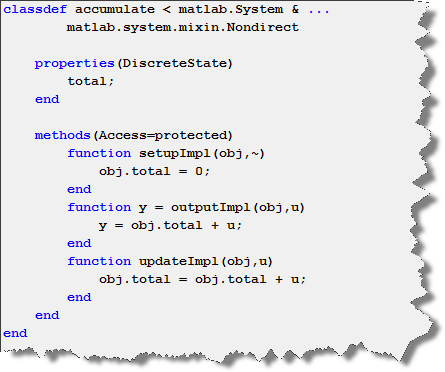# Methods of the MATLAB System Block

In the last post on MATLAB System block, I tried to provide a basic introduction to System Objects in MATLAB and Simulink environments. At the end, I finished with a quick example illustrating that System objects offer several methods to set up the properties of states and output signals of the system developed.

This week, guest blogger Revathi Dukkipati will help us get a closer look at System object methods and the sequence in which they are implemented.Timing of methods in MATLAB

When authoring System objects, one important thing to realize is that there are methods that you can call (for example step to process data), and the methods that you implement (for example stepImpl when you write your algorithm).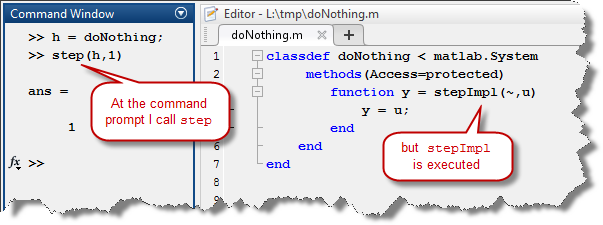To understand why there is a difference between the name of the method you call and the name of the method you implement, I recommend looking at the Methods Timing documentation page. You will find images like the following illustrating which methods you can call, and which methods you can or need to implement.

For the step method, this looks like: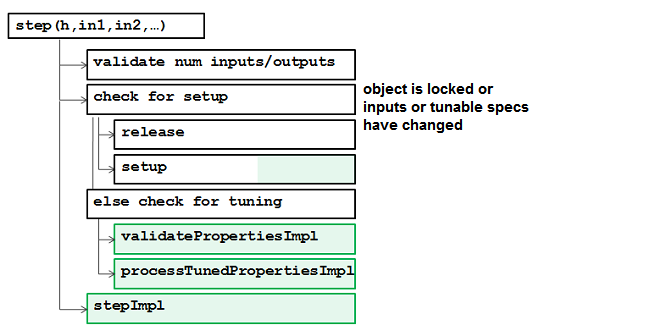The methods shown with a green background can be implemented by the user. Except for stepImpl which implements the algorithm, the other methods in green have an internal default implementation. You only need to implement them if the default is not appropriate for your application. The methods shown in white are completely controlled by MATLAB.

When using a System object in Simulink through the MATLAB System block, it is important to know which methods are called during edit time, which methods are called during the model compilation and which methods are called every time step when the simulation runs.

You can find an overview of this timing in the documentation page Simulink Engine Phases Mapped to System Object Methods: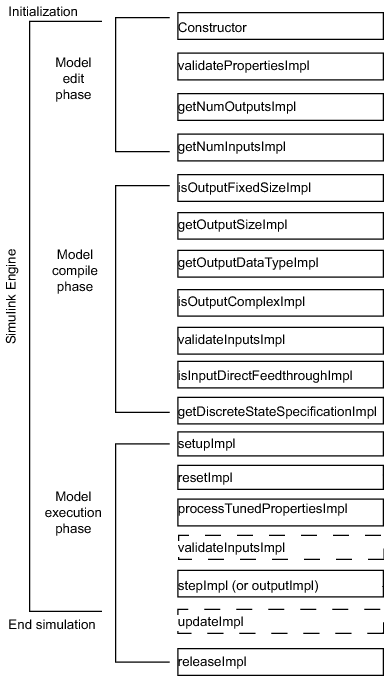Let's look at those phases in more details with example.:

Model Edit phase: This is where the object is created. Methods like getNumOutputsImpl and getNumInputsImpl need to be called for the Simulink editor to know the number of outputs and inputs of the block. Those methods are optional, you need to implement them only if your block has more than one input port and one output port.

Here is an example System object with two inputs and three outputs: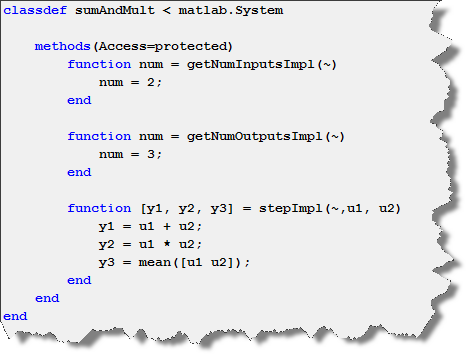Model Compile phase: When simulating a model, before the simulation can start, Simulink needs to determine the dimensions and other properties of all signals. For the properties of its input ports, the MATLAB system block inherits them from the blocks connected to it. For the output ports, the Simulink engine can try to infer them from the input ports, or call the propagation methods of your System object.

These methods are inherited from the base class matlab.system.mixin.Propagates and required when the output specifications cannot be inferred directly from the inputs. Even when output specifications can be inferred, adding the Propagation methods can improve the compilation time of your model since the time spent on inferring this data is saved.

Here is an example where the output has a dimension of five: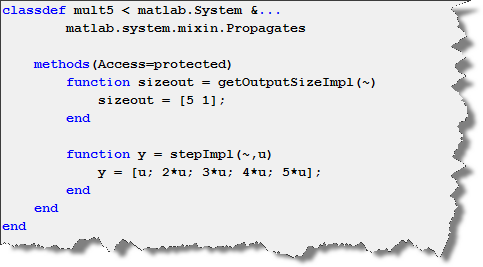Model Execution phase: Once the model initialization is completed, the algorithm in stepImpl is called at every step. If the block contains states, I recommend separating the stepImpl into two functions: outputImpl where you compute the values of output signals, and updateImpl where you update the values of states internal to the block. Those two methods are provided by matlab.system.mixin.Nondirect class.

Here is an example implementing an accumulator, using outputImpl and updateImpl